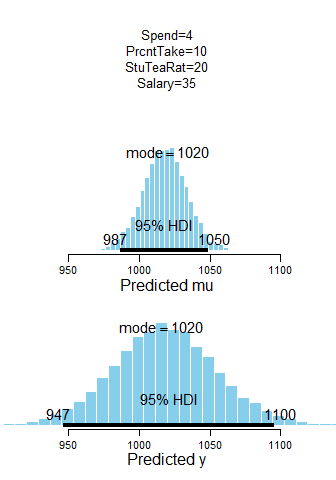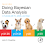## Sunday, June 11, 2017

### Posterior distribution of predictions in multiple linear regression

In a previous post I showed how to compute posterior predictions from multiple linear regression inside JAGS. In this post I show how to do it after JAGS, in R.

There are trade-offs doing it in JAGS or afterwards in R. If you do it in JAGS, then you know that the model you're using to generate predictions is exactly the model you're using to describe the data, because you only specify the model once. If you do it after JAGS in R, then you have to code the model all over again in R, and face the peril of making mistakes because JAGS and R parameterize distributions differently (e.g., in dnorm and dt are parameterized differently in JAGS than in R). On the other hand, if you generate the predictions in JAGS, then you have to specify all the to-be-probed x values in advance, along with the data to be fit. If instead you generate predictions in R (after JAGS), then you can re-use the already-generated MCMC chain for to-be-probed x values as much as you like, without having to run MCMC all over again. In this post, we face the peril of specifying the model in R.

Please see the previous post for background information about this specific application to predicting SAT scores from spending per student and percentage of students taking the exam. To run the R code below, please open the high-level script Jags-Ymet-XmetMulti-Mrobust-Example.R. Run the script "out of the box" so it uses the SAT data (Guber1999data.csv) and the two predictors mentioned in the previous sentence. Then, at the end of the script, append the following R code:

# Remind self of the predictors:
show( xName )
# Specify probe values of the predictors. Columns must match predictors actually
# used, in order! Specify as many probe rows as you like:
xProbe = matrix( c( 4.0 , 10 ,   # Spend , PrcntTake
9.0 , 10 ,   # Spend , PrcntTake
4.0 , 90 ,   # Spend , PrcntTake
9.0 , 10 ) , # Spend , PrcntTake
ncol=length(xName) , byrow=TRUE )
# Convert mcmc coda object to matrix:
mcmcMat = as.matrix( mcmcCoda )
# Show column names of mcmcMat. Notice they include beta0, beta, beta,
# ..., which can be extracted using grep("^beta",colnames(mcmcMat)):
colnames( mcmcMat )
grep("^beta",colnames(mcmcMat),value=TRUE)
# Now, go through rows of xProbe matrix and display predicted mu and y:
for ( xProbeIdx in 1:nrow(xProbe) ) {
# Predicted mu is b0+b1*x1+b2*x2+... at every step in chain:
muPred = ( mcmcMat[,grep("^beta",colnames(mcmcMat))]
%*% matrix(c(1,xProbe[xProbeIdx,]),ncol=1) )
# Predicted y is is pred mu plus t-distrib noise at every step in chain:
yPred = muPred + mcmcMat[,"sigma"] * rt(nrow(mcmcMat),df=mcmcMat[,"nu"])
# Make plot of posterior distribution of prediction. Notice that plotPost()
# also returns numerical values to console.
openGraph(width=3.5,height=5)
layout(matrix(1:3,nrow=3),heights=c(1.5,2,2))
par( mar=c(4,1,1,1) , mgp=c(2.0,0.7,0) , xpd=NA )
plot(0,0,xaxt='n',yaxt='n',bty='n',pch='',ylab='',xlab='')
paste0(xName,"=",xProbe[xProbeIdx,],collapse="\n"), cex=1.25 )
xLim = quantile( yPred , probs=c(0.005,0.995) )
muPredInfo = plotPost( muPred ,xlab="Predicted mu" , xlim=xLim ,
main="" )
show( muPredInfo )
yPredInfo = plotPost( yPred , xlab="Predicted y" , xlim=xLim ,
main="" )
show( yPredInfo )

The code produces output plots such as the following (along with numerical values displayed on the command line):N.B.: The plots only display the first three significant digits; see the numerical output at the command line for more digits.

Enjoy!

Reminders of some recent posts:

1.If you happened to view this post within its first day, you'll have seen R code that had a couple vestigial lines that only worked for TWO predictors instead of any number of predictors. I modified the code so it now works with any number of predictors. The updated lines are these:

for ( xProbeIdx in 1:nrow(xProbe) ) {

# Predicted mu is b0+b1*x1+b2*x2+... at every step in chain:

muPred = ( mcmcMat[,grep("^beta",colnames(mcmcMat))]

%*% matrix(c(1,xProbe[xProbeIdx,]),ncol=1) )

2.I just made another modification/correction to the originally posted code. The originally posted code had an error in the plotting at the end, in which the `main' argument referred to xProbe1, a variable that didn't exist. The code is now corrected so the plots display the x probe values for any number of predictors.

3.Thanks for posting this! It would be amazing to see an explanation of how to find the posterior distributions of predictions for a coupled model. I'm thinking of a zero-inflated Poisson where the zero-inflation is modeled by a binomial distribution. I'm stuck on how to simulate y when it's generated by multiplying lambda (which I can find by using the parameters at probed values of x) by the binomial variable, w[i], to get an effective lambda that models the Poisson count. That extra step, w[i], is throwing me off.

I know I can't be the only person with this problem!

Thanks for all your great work and for the great blog!

1.At step s in the MCMC chain, use lambda[s] inside rbinom() or rpois() to generate the predicted count.

If the count probability is a weighted mixture of binom and pois, then choose the result of rbinom() or rpois() with that probability. For example, if it's 0.33 * binom + 0.67 * pois then let
w = runif(0,1)
and
if ( w <= 0.33 ) { y=rbinom(lambda[s]...) } else { y=rpois(lambda[s]...) }

2.Thank you for your reply and suggestion! This has put me on the right path to working out a solution, and has helped me to understand what's going on more generally when we find the posterior distribution of the predictive value.